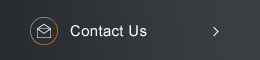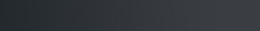Sponsored by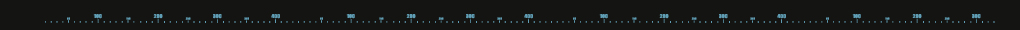[ Events ]

﻿
Activity Search
Sort out
 select all Seminars and Talks Conference and Workshop Lectures and Courses Else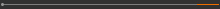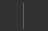Field
 select all Number Theory and Representation Theory Algebraic Geometry Differential Geometry and Geometrical Analysis Differential Equations and Stochastic Analysis Scientific Computing Interdisciplinary Studies Laboratory of Data Science Harmonic Analysis OthersYear
 select 2015 2016 2017 2018 2019 2020 2021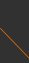Nonlinear Phenomena in Evolutionary Partial Differential Equations15:30 - 17:30, May 21, 2019 (Tuesday) R537, Astronomy-Mathematics Building, NTU (台灣大學天文數學館 537室) Introduction to Einstein-Boltzmann System Yuan-Chieh Chen (National Taiwan University) Abstract: A Einstein-Boltzmann system is the Einstein equations coupled with the Boltzmann equation, where the stress-energy tensor in the Einstein equations is described by the Boltzmann equation and the distribution function describing dynamics of relativistic gas determined by the Boltzmann equation is defined on the four dimensional manifold whose metric is given by the Einstein equation. In this talk, I'll discuss the existence and asymptotic behavior of solutions of the Einstein-Boltzmann system in spatially homogeneous space-time.(C) 2019 National Center for Theoretical Sciences